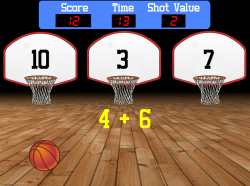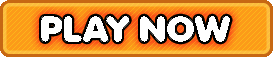Bookmark

# Shot Clock Basic Math Facts Addition, Subtraction, Multiplication, Division

4.6 stars (3,243 plays / 23 votes)Shot Clock | Basic Math Facts (Addition, Subtraction, Multiplication, & Division)Shoot the basketball into the hoop showing the correct answer.
4.6 stars out of 5 (3,243 plays / 23 votes)

KidzSearch > Games > Math GamesTags:  math    basic    fact    addition    subtraction    multiplication    multiply    division    divide    timed    math    problem    elementary    math    number    sense    sportsMember of the American Library Association  Copyright 2005-2020 KidzSearch.com